# What are geometric shapes? Know everything from scratch

“Geometric shapes” is a term that we are familiar with since a very young age. Since early in the classroom, we have learned the various geometric shapes in math. So, what most of us know are geometric shapes of three dimensions. However, out of these, only a straight line would be a 1-D geometric shape. We are most familiar with 2-D geometric shapes. So, these include the triangle, the square, the rectangle, the circle, the parallelogram, the rhombus, the hexagon, the heptagon, the octagon, the nonagon, the decagon, and so on till infinity. There are 3-D geometric shapes as well. So, here we have the cube, the cuboid, the sphere, the cylinder, the cone, and so on.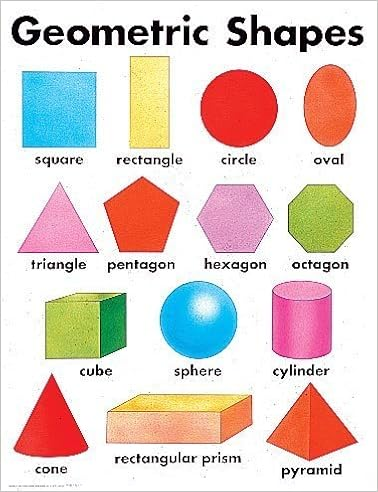Now, while doing math at school, we mostly use 2-D geometric shapes. However, if you think properly, it is the 3-D shapes that we see regularly around us. So, it makes sense because we do not live in a 2-D world. So, a matchbox is cuboid and not rectangular.

However, these are just examples we know about geometric shapes. But, it is important to know their definition and meaning along with examples.

## Geometric shapes definition with examples

The best possible way to understand geometric shapes is to link definitions with examples. This is because otherwise you may know what geometric shapes are in your head but you cannot express them with pen and paper. Examples, on the other hand, are important because the expanse of geometric shapes is vast. So, a general definition cannot cater to every kind of geometric shape. Hence, let us see what geometric shapes mean at first.

## Geometric shapes meaning

So, geometrical shapes are the figures which represent the forms of different objects. As we already know, such figures can be two-dimensional, whereas or three-dimensional. So, now, the two-dimensional figures lie on only the x-axis and y-axis. However, the 3-D shapes lie on the x, y, and z axes. So, the z-axis is the addition in 3-D figures. It shows the height of the object. Moreover, the 2-D figures are also known as flat shapes or closed shapes. But, there are various shapes under these expansive “geometric shapes” which represent the shapes of various objects around us.Geometric shapes, moreover, can be of two types. So, they may be regular or symmetrical. This means that all the sides in this shape are either equal or share a fixed ratio. On the other hand, they may be asymmetrical or irregular as well. Here, the lines forming the geometric shape might go haywire. So, mathematicians also call these organic shapes.

Now, if you want to draw or design any of these figures, you must start with a line, or a line segment, or a curve. So, we get different types of shapes and figures like a triangle, a figure where three line segments are connected, a pentagon (five-line segments), and so on. This depends on the number and arrangement of these lines. However, we should also remember that every figure is not a complete figure. Now, let us see a few examples to amplify the idea.

## Geometric shapes examples

First, we must know what open and closed shapes are. So, to draw an open shape you must have a separate initial and ending point that must not coincide. Therefore, what this means is that your line is a regular, or irregular, straight or curved line that does not enclose a space. On the other hand, if the initial and endpoints coincide, they enclose a space. So, they form a closed shape. Circles, squares, rectangles, and so on are all examples of closed shapes. So, we will go through their properties and formulas in the next few sections.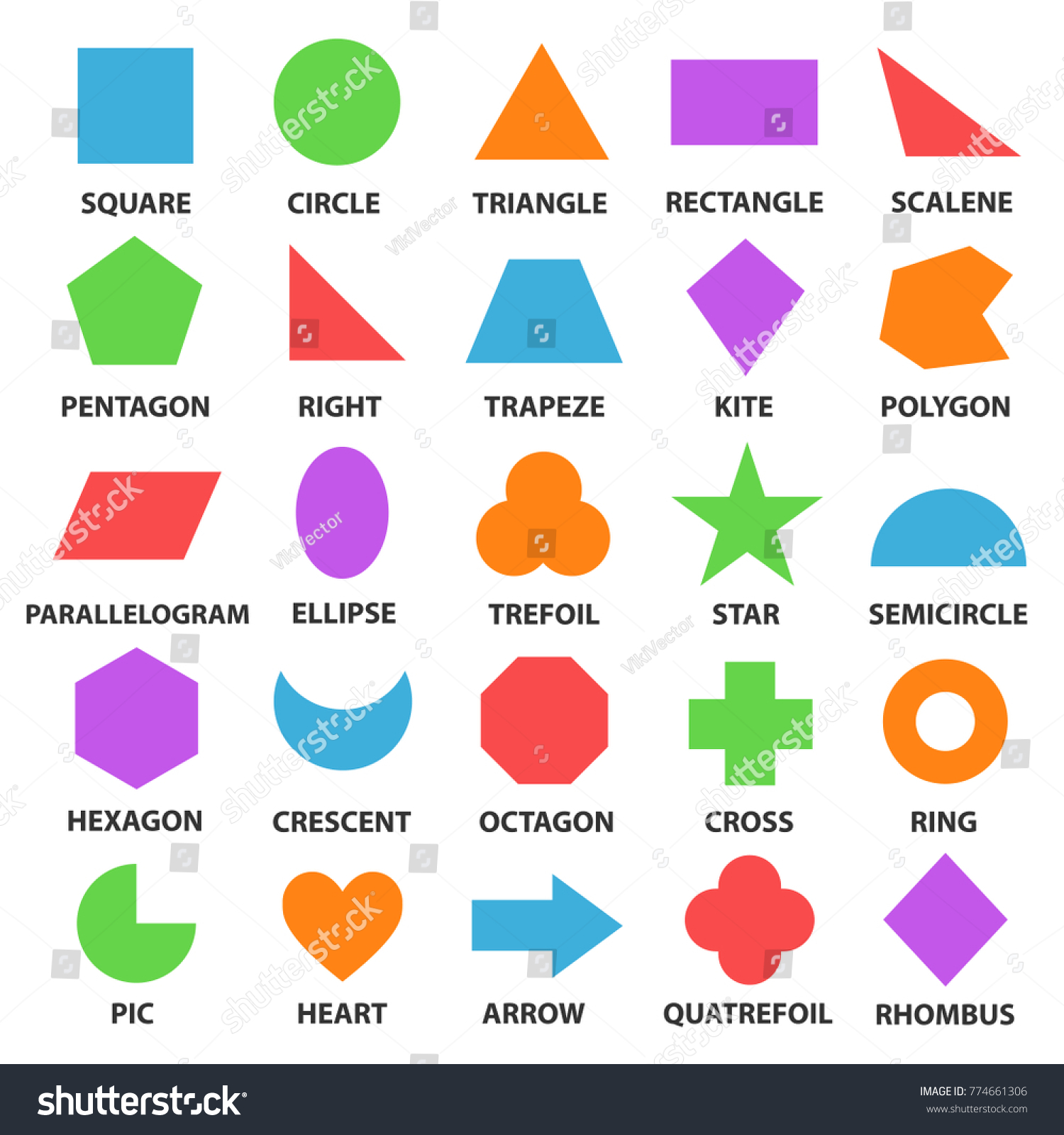## Geometric shapes and their properties

So, as we have already seen, the expanse of geometric shapes is huge. Therefore, various figures fall under them. As one can well imagine, each of these figures must have its own set of properties because they are totally different shapes. However, you need to remember that you can properly state the properties of only regular geometric shapes that you know about. You cannot define irregular shapes by any of their properties as such. Let us look at a few of them.

## Geometric shapes triangle

Triangles are 2-D geometric shapes. So, it is a polygon that has three sides. Moreover, it also has three edges and three vertices. However, the most important thing that you must remember regarding triangles is that the sum of their internal angles must be equal to 180 degrees.## Geometric shapes squares

Squares are probably the most basic geometric shapes. They are two-dimensional. So, they have four sides and all of them are equal in length. Moreover, all the angles are equal to cutting the sides at perfect 90 degrees.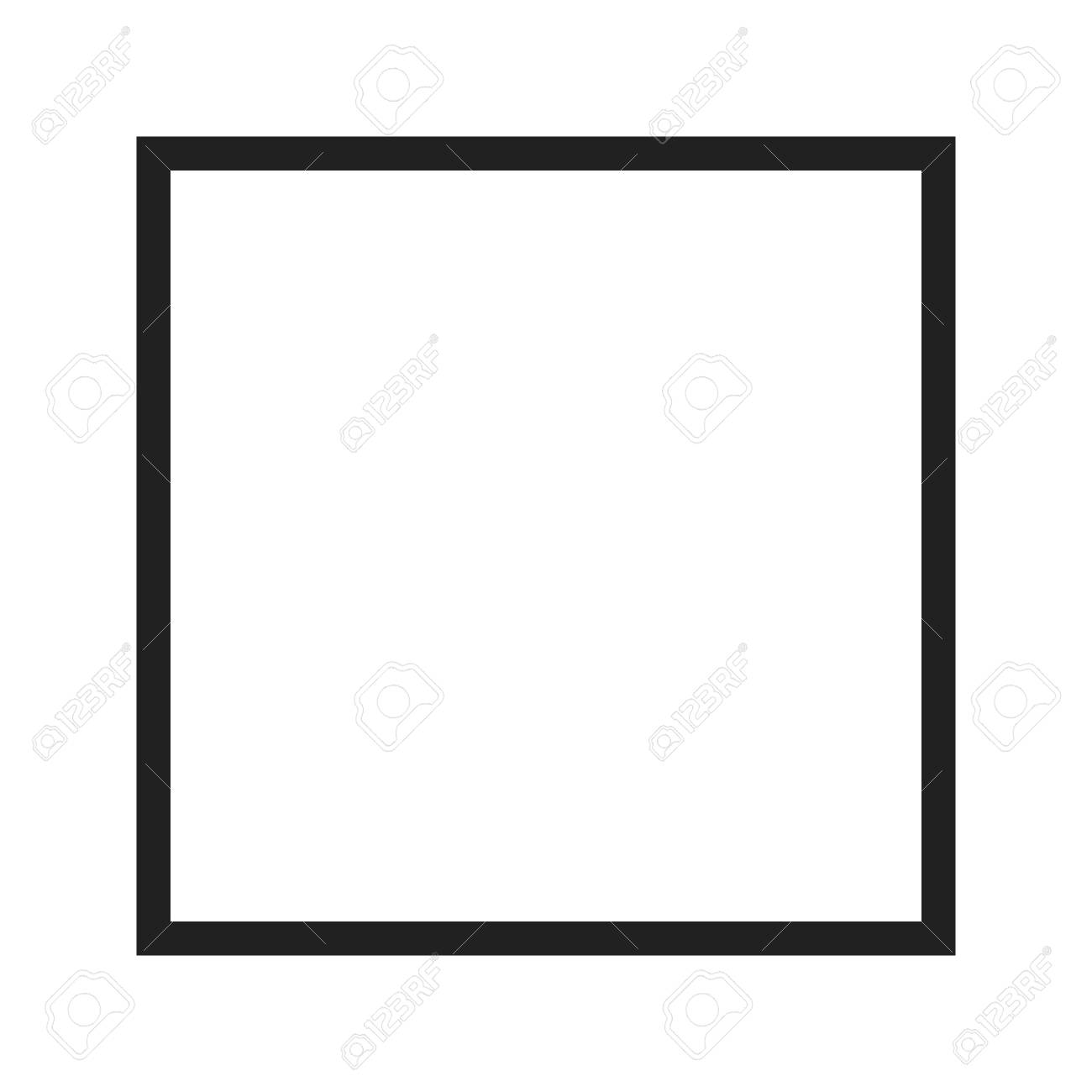## Geometric shapes rectangle

2-D shapes like squares and rectangles that have four sides are called quadrilaterals. This is because Quadri means 4 and laterals mean lines. So, these geometric shapes have four lines that join with each other to form them. So, a rectangle also has 4 sides with vertices at 90 degrees. However, it is different from a square. This is because, in a rectangle, only the opposite sides are equal.## Geometric shapes rhombus

These are also 2-D geometric shapes. However, it is quite easy to define them. A rhombus is just a square without necessarily 90-degree vertices. So, it is also a quadrilateral. Therefore, it is a parallelogram with all equal sides. Parallelograms are quadrilaterals that have two pairs of parallel sides. However, their opposite angles are also equal in measurements.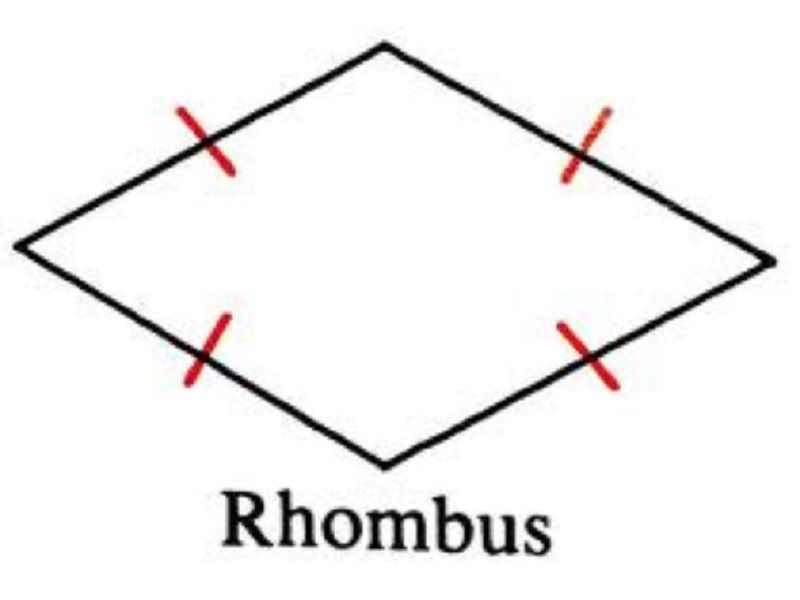## Geometric shapes circle

So, circles are geometric shapes where the locus of all points are at a fixed distance from a reference central point. Therefore, this central point is the center of the circle. On the other hand, the movement of the loci forms the circumference of the geometric shape. The fixed distance between the center of a circle and a locus is called the radius. Moreover, the distance between two opposite loci touching the center is the diameter of the circle. It is a 2-D figure.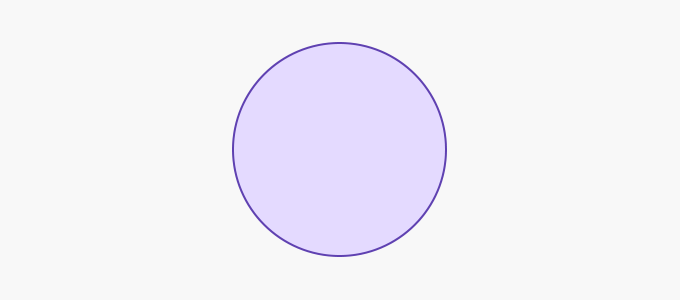## Geometric shapes cube

So, cubes are 3-D geometric shapes. However, all the sides of a cube are exactly the same. So, a cube has 6 faces, 8 vertices, and 12 edges. Since all the sides are equal in size, each face of a cube is a square.## Geometric shapes cuboid

Cuboids are again 3-D geometric shapes. They too have 6 faces, 8 vertices, and 12 edges. However, they are different from cubes. This is because each face of a cuboid is a rectangle. So, the length, breadth, and height all are of different proportions.## Geometric shapes cone

So, we have all seen ice-cream cones. The geometric shapes that they represent are cones. So, they are definitely 3-D. They have a circular base. Moreover, the sides narrow down from the base to the top like a triangle. So, at the top, they form a point. We call this the apex or the vertex.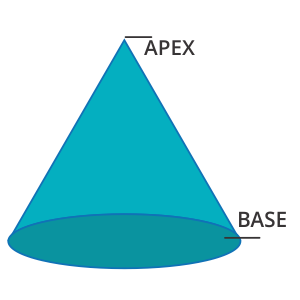## Geometric shapes cylinder

Now, cylinders are 3-D geometric shapes that have no vertex. So, they have two circular bases that are parallel to each other. Now, a curved surface connects these two bases.### Geometric shapes polygon

So, these are geometric shapes that have only line segments and no curves. They are different closed figures. So, they depend on different lengths of sides and different angles. Therefore, geometric shapes like squares, rectangles, hexagons, octagons, pentagons, heptagons, and so on are all polygons.

So, now that we have more or less seen the properties of major geometric shapes, let us go through the formulas.

### Geometric shapes and their formulas

Basic formulas of geometric shapes:

The perimeter of a square = 4a, where a is each side of these geometric shapes.

So next, the perimeter of a rectangle = 2 x (l + b), where l is the length and b the breadth of these geometric shapes.

Next, the perimeter of a triangle = a + b + c, where a, b, and c are the three sides of the triangle. So, for an equilateral triangle, all these sides are the same. For an isosceles triangle, two sides are equal while for a scalene triangle, all three must be different.

Therefore, next comes a circle. We do not call the perimeter of a circle because it is not a line. So, the circumference of a circle is 2 ? r where r is the radius of the circle.

Hence, we have seen the basic formulas of geometric shapes. Now, let us deal with the complex area or volume formulas of both 2 and 3-D geometric shapes.

### Geometric shapes and area formulas

So, let us begin with the 2-D geometric figures first.

Therefore, the area of a square is a^2 where a is each side of these geometric shapes. Similarly, the area of a rectangle is l x b where l and b are respectively its length and breadth. So next, the area of a triangle is ½ x b x h where b is the base and h is the height of the triangle. However, you might always not have the height of the triangle at hand. Moreover, if the triangle is scalene you cannot even apply the Pythagorean theorem. So, in those cases, you have to first find its semiperimeter. Then, you can apply Heron’s formula.

Read Also: Electric Force: Definition & Equation

So, finally, you have the circle. To find the area of the circle, you have to do ? r2 where r is the radius of the circle.

Now, let us go for the 3-D figures. These geometric figures would have a surface area instead of a simple area. Let us see them.

### Geometric shapes surface area

For a cube, the surface area is 6 a^2, where a is the length of each side. So next, for a cuboid, the surface area is 2 ( l x b + b x h + l x h), where l, b, and h are respectively the length, breadth, and height of the cuboid. Therefore, next, we have the surface area of a sphere. We can calculate this by 4? r2, where r is the radius of the sphere. Now, we have the cylinder and the cone. For these two geometric shapes, we can have a total and curved surface area. So, the difference between the total surface area and curved surface area is that the former considers only the sides while the latter considers both bases and sides.

Therefore, the curved surface area of a cylinder is 2 ? rh, and the total surface area is 2?r (r + h). So, here r is the radius of the base, and “h” is the height of the cylinder. Now, the curved surface area of a cone is ?rl and the total surface area is ?r (r + l). So, here r is the radius of the base and l is the length of each side. Furthermore, you can find out the value of l from h and r by using the Pythagoras theorem.

### Geometric shapes volume formulas

So now let us finally see the formulas that will help us find the volumes of 3-D geometric shapes. 2-D geometric shapes cannot have volume formulas because they are planar. They only enclose an area and not a volume.

So, the volume of a cube is a^3 where a is the length of each side of the cube. Next, the volume of the cuboid is l x b x h where l, b, and h are respectively the length, breadth, and height of the cuboid. Now, the volume of a sphere is 4/3 ? r^3 where r is the radius of the sphere. Now, the volume of a cone is ⅓ ? r^h where r and h respectively are the radius of the base and the height. Finally, the volume of a cylinder is ? r^h where r and h respectively are the radius of the base and the height.

### Geometric shapes edges vertices faces

So, these are some of the very basic terms that we encounter while discussing geometric shapes. Let us quickly see what each of these terms means in short.

So, edges in geometric shapes are a particular type of line segment joining two vertices in a polygon, polyhedron, or any higher-dimensional polytope. In the case of a polygon, an edge is a line segment on the boundary. So, we often call it a polygon side.

Therefore, the next thing we must know is vertices. Vertices are the plural of vertex which are the corners in the geometric shapes. So, a vertex is a meeting point of two lines or edges in any polygon.

So, faces in solid geometry are flat surfaces or planar regions. Therefore, they form part of the boundary of a solid object. So, faces bounding a three-dimensional solid is a polyhedron.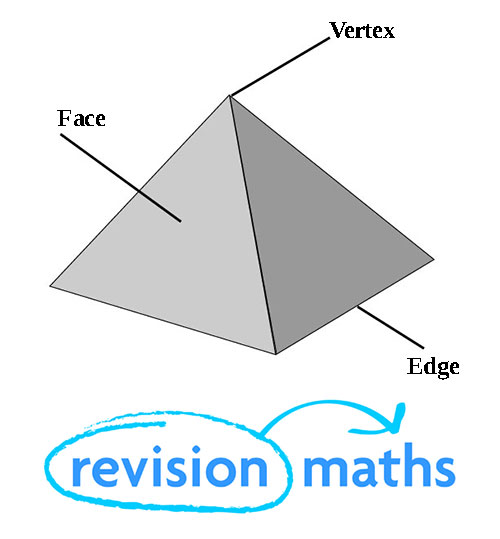### Geometric shapes characteristics

To sum up, roughly, geometric shapes have some very basic general characteristics-

• Geometric shapes can be of any structure.
• Each structure has a unique set of properties. However, structures may coincide, like all squares are rectangles but not the other way round.
• They may be open or closed.
• The lines and points decide their properties.

### What makes a geometric shape geometric?

Ans. This is a very valid question because how do we know which shapes are geometric shapes. So, a geometric shape is a piece of geometric information that remains when location, scale, orientation, and reflection are removed from the description of a geometric object.

Ans. It finds a lot of importance regarding how we describe or want something to be. For example, you go to the carpenter’s shop to get an almirah, you need to tell its geometric shape and dimensions. However, the most important use is perhaps architecture and civil engineering- from the shape of an engine to the shape of the ventilators in your home.

### Are geometric shapes 2d and 3d?

Ans. As we have already seen, geometric shapes can be both 2-d as well as 3-d. It depends on what figure we are considering. 2-d shapes occupy an area. On the other hand, 3-d shoes occupy a volume in the space.

### Who invented geometric shapes?

Ans. Euclid invented most of the geometric shapes. He was one of the greatest mathematicians of all time. Moreover, he is also the father of geometry.### What are geometrical instruments?

Ans. Geometrical instruments are key to learning geometry. This is because figures need to be of the most precise dimensions. So, there are various devices for drawing geometric shapes like rulers, protractors, compasses, dividers, and so on.

### Which geometric instrument is used to construct a square?

Ans. Constructing a square is pretty basic. So, you will need a ruler and a half-circle protractor for this. First, draw the baseline with the ruler. Then, draw a 90-degree protractor (you can also do this by a compass) on one side of the base. Repeat the same thing on the other side. So, measure the base and cut equal length on both sides. Join these two with another line. So, you have your square ready.

### What is the difference between organic and geometric shapes?

Ans. So, organic shapes are curvilinear in nature. They occur around us but do not have any particular definition- like the shape of a rock which can always vary. On the other hand, geometric shapes have a constant definition and must have principles that pure mathematics can define.

### Which is the geometrical instrument used to draw a circle?

Ans. Drawing a circle is probably the easiest thing to do in geometry. So, you can easily do this with a pencil and a pair of compasses.### Is a star a geometric shape?

Ans. Yes. Any 2-D closed shape with lines is a polygon. Therefore, a star is a regular polygon. So, it is a geometric shape.

### When was geometry invented?

Ans. In all probability, the Greeks invented it sometime around the 6th century BCE. It is therefore a combination of the Greek word geo meaning earth and metron meaning measurement.